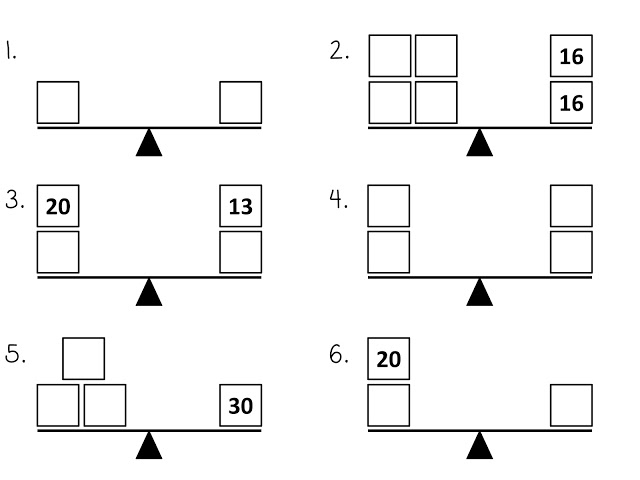Match Fishtank is now Fishtank Learning!

# Solving One-Variable Equations

## Objective

Understand that equations can have no solutions, infinite solutions, or a unique solution; classify equations by their solution.

## Common Core Standards

### Core Standards

?

• 8.EE.C.7.A — Give examples of linear equations in one variable with one solution, infinitely many solutions, or no solutions. Show which of these possibilities is the case by successively transforming the given equation into simpler forms, until an equivalent equation of the form x = a, a = a, or a = b results (where a and b are different numbers).

?

• 7.EE.B.4

## Criteria for Success

?

1. Understand that if an equation has no solution, then there is no value for x that will make the equation true; for example ${ x+2=x+3}$ has no solution.
2. Understand that if an equation has infinite solutions, then $x$ can represent any value and it will make the equation true; for example $x+1=x+1$ has infinite solutions.
3. Understand that if an equation has a unique solution, then there is one value for $x$ that will make the equation true; for example $x+1=3$ has a unique solution of $x=2$.

## Tips for Teachers

?

Lessons 8 and 9 introduce students to the three types of solutions that an equation can have. This lesson focuses on students understanding what each solution means about the equation, and on becoming familiar with arriving at solutions that look like ${4=2}$ or ${-8=-8}$ (MP.2). In the next lesson, students will reason with all three types of solutions to further internalize what they mean. The concept of no solution, infinite solutions, and unique solution will re-appear when students study systems of linear equations in Unit 6.

#### Remote Learning Guidance

If you need to adapt or shorten this lesson for remote learning, we suggest prioritizing Anchor Problem 1 (benefits from discussion) and Anchor Problem 2 (benefits from worked example). Find more guidance on adapting our math curriculum for remote learning here.

#### Fishtank Plus

• Problem Set
• Student Handout Editor
• Vocabulary Package

## Anchor Problems

?

### Problem 1

These scales are all currently balanced. You must choose a number to fill into the boxes in each problem that will keep them balanced. In each individual box you may only use one number.#### References

Everybody is a Genius Solving Special Case Equations

Solving Special Case Equations is made available on Everybody is a Genius under the CC BY-NC-SA 3.0 US license. Accessed Aug. 31, 2017, 2:41 p.m..

### Problem 2

Sort the equations below into the three categories.

a.   ${3x=0}$

b.   ${3x=2x}$

c.   ${3x=2x}+x$

d.   ${2x+1=2x+2}$

e.   ${3x-1=2x-1}$

f.   ${x+2=2+x}$

g.   ${x+2=x-2}$

h.   ${2x-3=3-2x}$

 Infinite Solutions Unique Solution No Solution

### Problem 3

Solve the equations below and explain what each solution means.

a.    ${5x-2\left ({1\over2}+x \right ) + 8 = - {1\over4}(16-12x)}$

b.    ${1.6(x+4)-3(0.2x+1)=2x-{1\over2}(18.6)}$

c.    ${{{{1\over2}x +4}\over-1} = {{-x-8}\over2}}$

## Problem Set

?The following resources include problems and activities aligned to the objective of the lesson that can be used to create your own problem set.

?

Todd and Jason both solved an equation and ended up with this final line in their work: ${{-2}x=4x}$.

• Todd says, “This equation has no solution because ${{-2}\neq 4}$.”
• Jason says, “The solution is ${-2}$ because ${-2}({-2})=4$.”

Do you agree with either Todd or Jason? Explain your reasoning.

?